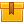# Pressure Measurement (AFM01)

## Prerequisites

This lesson is designed for participants familiar with basic mathematical operations including algebra.

## Description

This lesson presents the basic principles of pressure measurement and applications of direct and inferred pressure measurement methods. Various pressure instruments are presented including manometers, mechanical pressure sensors, and transducers.

## Objectives

Recognize the importance of the measurement and control of pressure. Define pressure and determine the pressure exerted by a liquid. Recognize the importance of sensor location in pressure measurement, calculate the force exerted by liquids. Identify the effects of temperature change on the force exerted by a liquid. Identify the factors which determine the force exerted by a gas. Convert various units of pressure measurement to psig, psia, InH2O, and InHg using a conversion table. Calculate differential pressure. Recognize the effect of atmospheric pressure on pressure measurement. State the principle of operation of closed and open manometers. Identify the types of manometers and the considerations for their safe and effective use. Describe the principle of operation for elastic elements. Explain how the movement of a sensing element can be used to produce a pneumatic and electrical signal. Describe the operation of mechanical to electrical transducers.

## ContentThis lesson presents the basic principles of pressure measurement and applications of direct and inferred pressure measurement methods. Various pressure instruments are presented including manometers, mechanical pressure sensors, and transducers.

•Pressure Measurement (AFM01)

0
0 Reviews